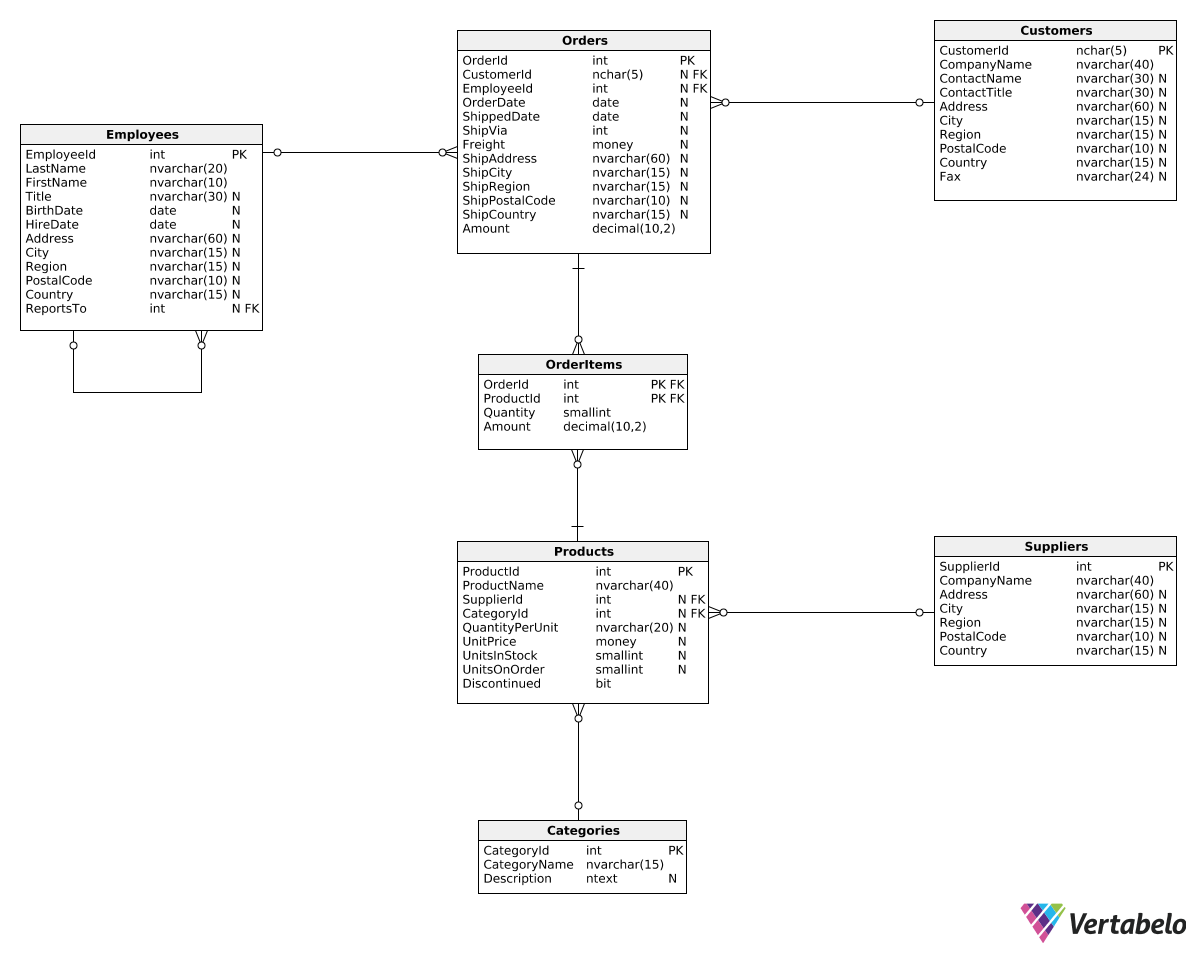Deals Of The Week - hours only!Up to 80% off on all courses and bundles.-Close
Introduction
Get to know the database
Basic revenue metrics
Summary

## Instruction

Now that we know the database, let's take a look at some basic revenue metrics.

In this part we'll discuss the following revenue metrics:

• Total revenue to date – the total revenue from all orders. We'll take advantage of this metric to get to know the revenue columns in our database.
• Total revenue in a period of time – often we're interested in the total revenue for a specific period of time, e.g., for a specific month or a specific quarter.
• Total revenue for the current year, month, etc. – they show how much the company earned so far in the given current period. Commonly used report types are year-to-date, quarter-to-date, or month-to-date.

The easiest metric to compute is the total revenue to date, i.e., the total revenue from all orders. Here is a SUM() function that will calculate it:

SELECT
SUM(Amount) AS TotalRevenue
FROM Orders;

You could also use the OrderItems table to get the same result:

SELECT
SUM(Amount) AS TotalRevenue
FROM OrderItems;

As you can see, we only need to sum all values from the Amount column of the Orders or OrderItems tables to get the revenue-to-date value. We can also add a WHERE clause to find the revenue-to-date for specific orders, for instance:

SELECT
SUM(Amount) AS TotalRevenue
FROM Orders
WHERE ShipCountry = 'USA';


The query above finds the revenue to date for orders shipped to the USA.

## Exercise

Find the total revenue to date for all beverages (i.e., products with CategoryId = 1). Use the Amount column from OrderItems as TotalRevenue.

### Stuck? Here's a hint!

Join the OrderItems table with the Products table and use SUM(Amount).